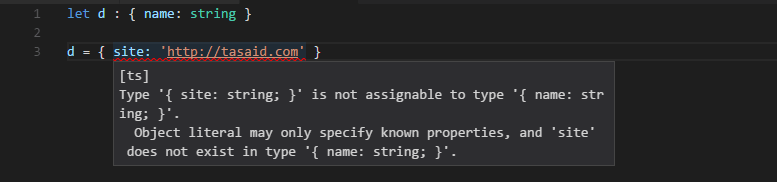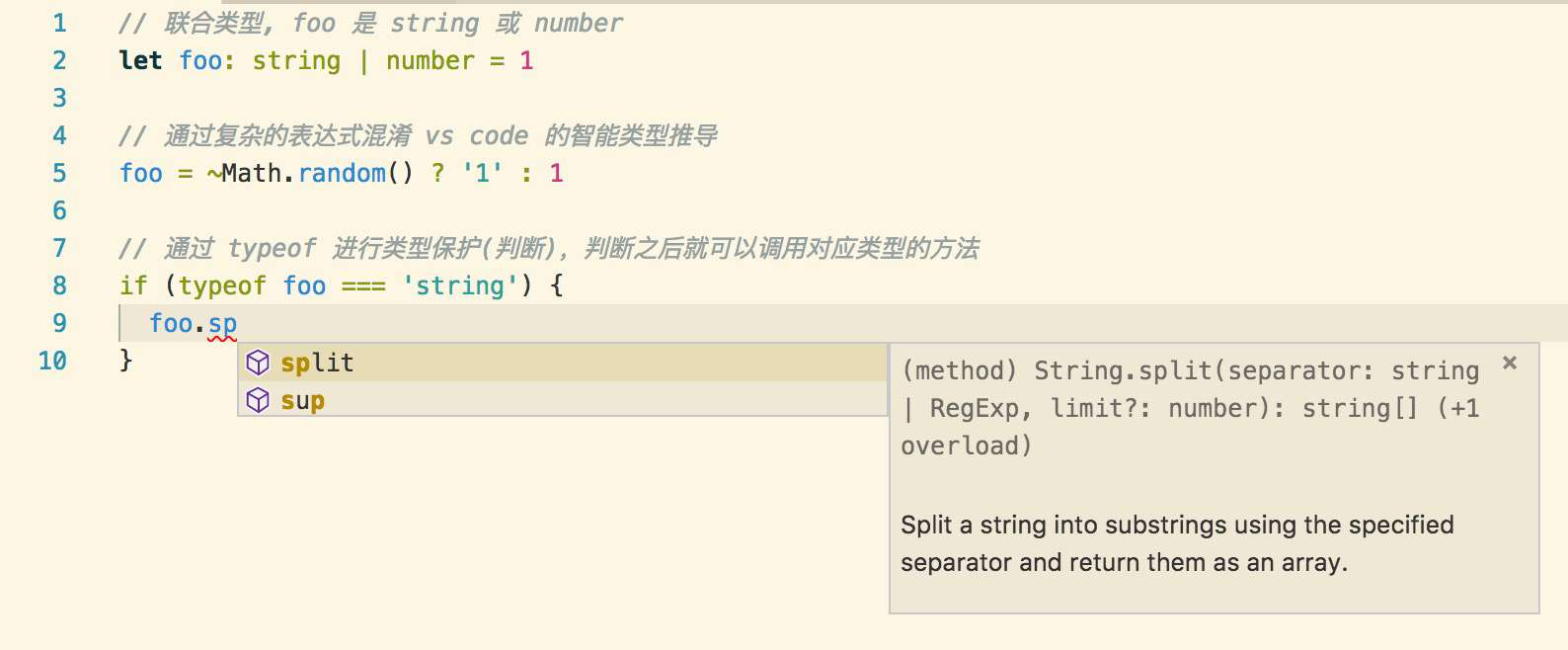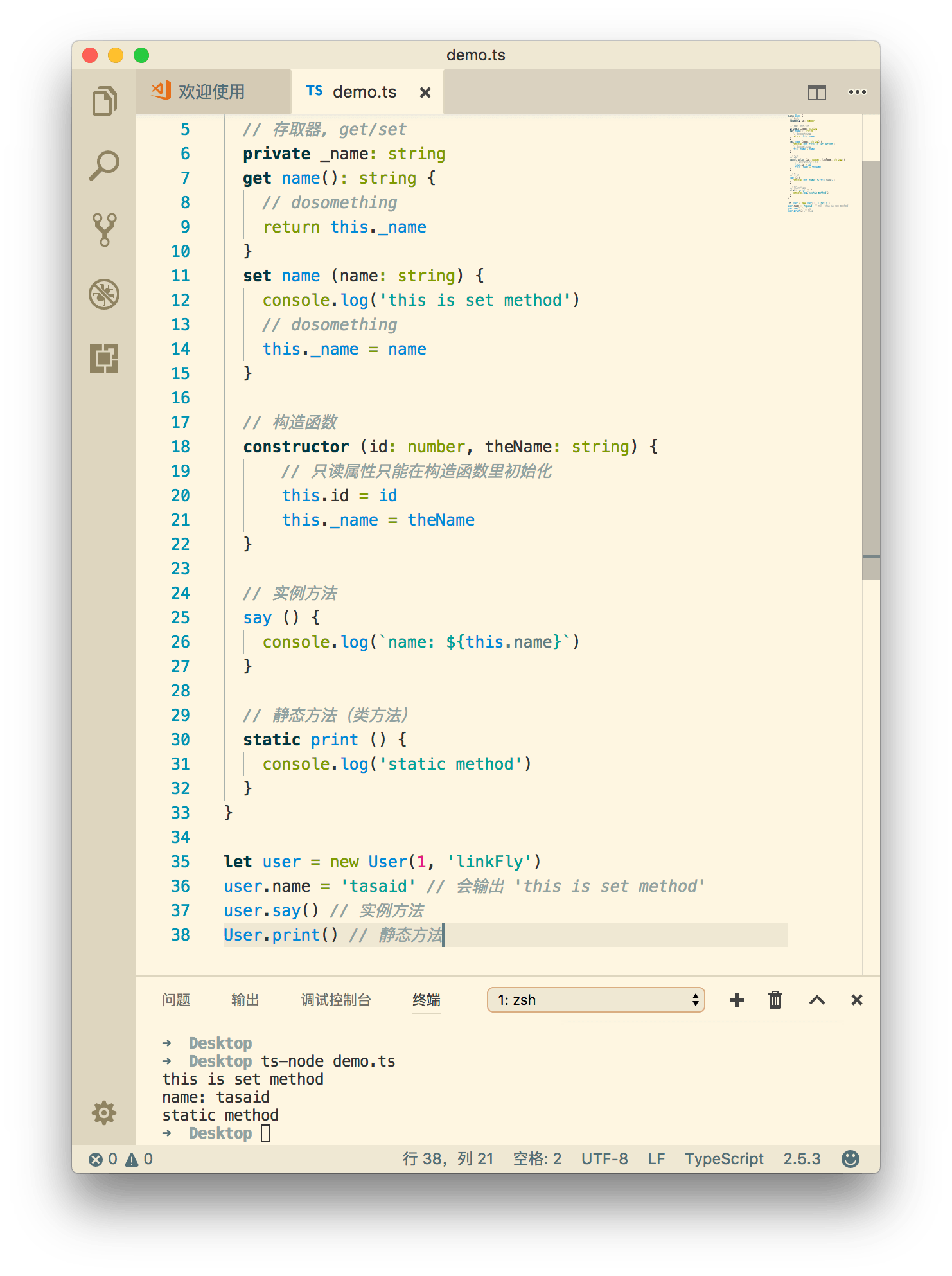# 从 JavaScript 到 TypeScript 2 - 基础特性和类型推导

## 基础类型

``````let a: number
let b = true  // 有默认值的情况，甚至不需要声明类型，ts 会自动推导
let c: [string, number] // 元组
enum Color {Red, Green, Blue} // 枚举
let d: { name: string } = { name: 'linkFly' }``````## 复杂类型

``````// array
let list_a: number[] = [1, 2, 3]
let list_b: Array<number> = [1, 2, 3] // number 类型的数组
let list_c: [string, number] = ['linkFly', 0]

// any
let notSure: any = 4
notSure = true // any 类型可以自由赋值

// 函数类型
let fn: (id: string) => number = (id) => 1
// 这里使用了 ECMAScript 6 的箭头函数，和下面的代码等价
let fn: (id: string) => number = function (id) {
return 1
}``````## 高级类型

``````// 联合类型, foo 是 string 或 number
let foo: string | number = 1

// 类型断言，强制使用兼容类型中的某一类型
(foo as string)

// 类型保护(判断)
if (typeof foo === 'string') {
// dosomething
}
// 类型保护(判断)
if (foo instanceof String) {
// dosomething
}``````## Model

``let user : { id: number, name: string } = { id: 1, name: 'linkFly' }``

### Class 类

``````class User {
id: number
name: string
}

let user: User = { id: 1, name: 'linkFly' }``````

``````class User {
// 只读属性

// 存取器, get/set
private _name: string
get name(): string {
// dosomething
return this._name
}
set name (name: string) {
console.log('this is set method')
// dosomething
this._name = name
}

// 构造函数
constructor (id: number, theName: string) {
// 只读属性只能在构造函数里初始化
this.id = id
this._name = theName
}

// 实例方法
say () {
console.log(`name: \${this.name}`)
}

// 静态方法（类方法）
static print () {
console.log('static method')
}
}

let user = new User(1, 'linkFly')
user.name = 'tasaid' // 会输出 'this is set method'
user.say() // 实例方法
User.print() // 静态方法``````### 泛型

``````function identity(arg: number): number {
// dosomething
return arg
}``````

``````// 这个 T 就是泛型，也可以叫其他名字
function identity<T>(arg: T): T {
// dosomething
return arg
}

identity(0)
identity(true)``````

``````function fetch<T>(url: string): Promise<T> {
// 远程请求数据并返回结果
return http(url).then(data => {
return data as T
})
}

class User {
name: string
}

// 泛型使用
let user = fetch<User>('https://tasaid.com/user')``````

## 小 tips

• TypeScript 中文网 可以看到完整 TS 类型。
• 在项目初期使用 TS 中，会需要很大的时间和精力，去编写和架构基本业务类型(Models)，在此之后会越来越方便快捷。
• 一些没有行为只需要做类型检查的类型（没有方法的 Models），可以使用 TypeScript 声明文件 （*.d.ts），例如：
``````declare namespace Models {
interface GPS {
lat: number
lng: number
}
}``````

• 尽量减少使用 `any` 类型，它意味着类型不可控
• 某些变量或者第三方库中属性无法感知，使用 `as` 强制进行类型推导即可。
``````window.tempName = 'linkFly'
// Errors: [ts] Property 'tempName' does not exist on type 'Window'.

// 强制推导

TypeScript 中文网：https://tslang.cn/

TypeScript 视频教程：《TypeScript 精通指南

11401
ce

fsd